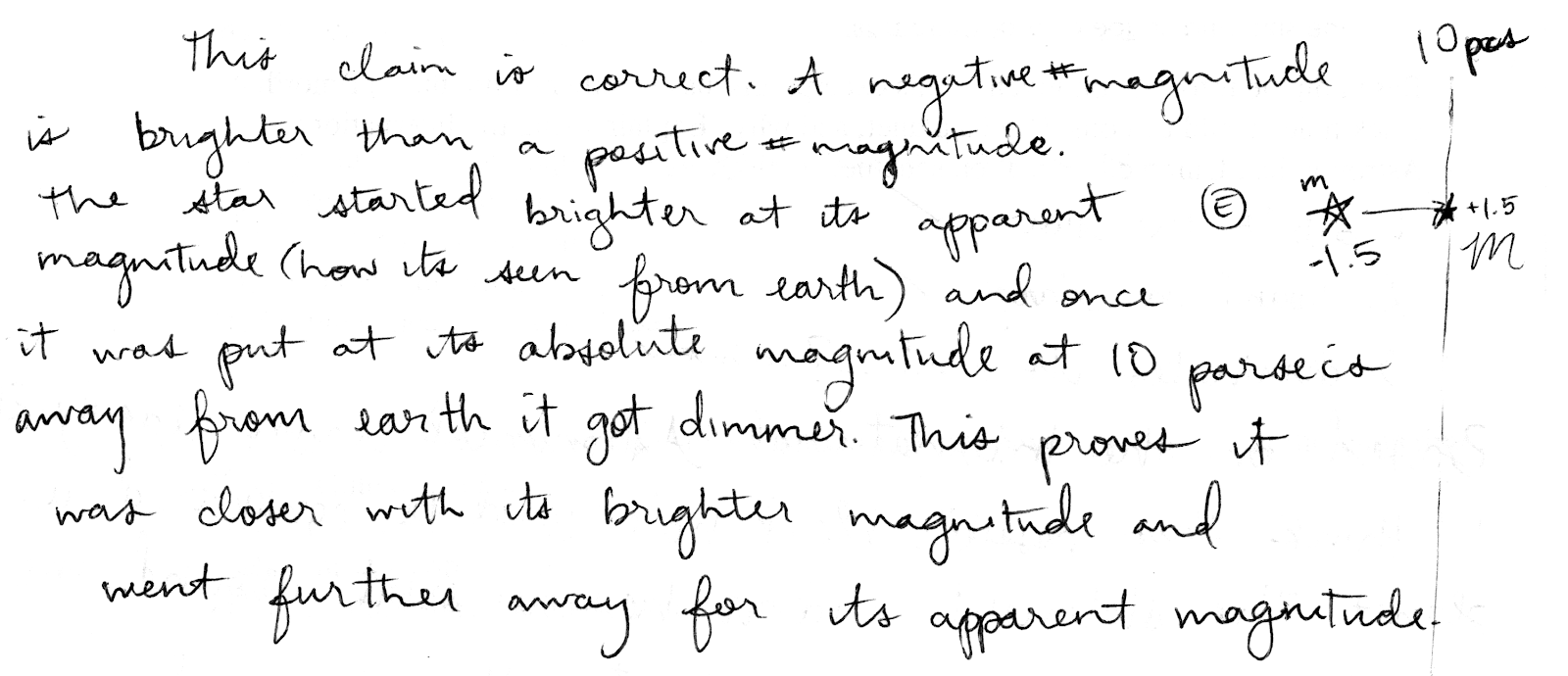## 20191122

### Astronomy midterm question: determining distance from apparent and absolute magnitudes (1)

Astronomy 210 Midterm 2, fall semester 2019
Cuesta College, San Luis Obispo, CA

The following claim was made by a student on an astronomy exam[*]:
0725: Let's say a star had an apparent magnitude of –1.5 and an absolute magnitude of +1.5. The star has to be closer than 10 parsecs from Earth.
Discuss whether this claim is correct or incorrect, and how you know this. Explain using the relationships between apparent magnitude, absolute magnitude, and distance.

[*] waiferx.blogspot.com/2008/11/astronomy-midterm-question-apparent.html.

• p:
Correct. Understands difference between apparent magnitude m (brightness as seen from Earth, while at its actual distance from Earth) and absolute magnitude M (brightness as seen from Earth, when placed at the "fair comparison distance" of 10 parsecs away), and discusses:
1. the star's apparent magnitude of m = −1.5 is brighter than its absolute magnitude of M = +1.5; so
2. the star must be located closer than 10 parsecs away, as moving it from its actual location (where m = −1.5) to 10 parsecs (where M = +1.5) makes it dimmer; such that
3. the student's claim is correct.
• r:
Nearly correct (explanation weak, unclear or only nearly complete); includes extraneous/tangential information; or has minor errors. Has both (1)-(2) complete and correct, but somehow concludes that student's claim is incorrect, or does not sufficiently discuss the correctness/incorrectness about the student's claim.
• t:
Contains right ideas, but discussion is unclear/incomplete or contains major errors. At least discussion demonstrates understanding of relationships between apparent magnitudes, absolute magnitudes, and distances. At least discussion demonstrates understanding of relationships between apparent magnitudes, absolute magnitudes, and distances. Has only one of (1)-(2) complete and correct, the other is problematic.
• v:
Limited relevant discussion of supporting evidence of at least some merit, but in an inconsistent or unclear manner. At least attempts to use relationships between apparent magnitudes, absolute magnitudes, and distances. Both (1) and (2) are problematic.
• x:
Implementation/application of ideas, but credit given for effort rather than merit. Discussion based on garbled definitions of, or not based on proper relationships between apparent magnitudes, absolute magnitudes, and distances.
• y:
Irrelevant discussion/effectively blank.
• z:
Blank.
Section 70158
Exam code: midterm02Rf0w
p: 23 students
r: 4 students
t: 1 student
v: 0 students
x: 6 students
y: 0 students
z: 0 students

A sample "p" response (from student 1492):